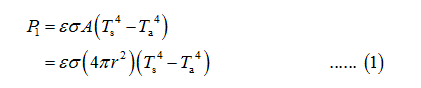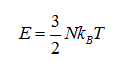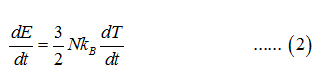# A steel spherical object is pulled from an oven at temperature 500C and placed on a surface in an environment at20C. Given the sphere's radius of 10cm, how long will it take to cool to 400C via blackbody radiation, which will beits primary means of losing heat? How long will it take if the radius is only 5cm? Explain the variation.

Question
10 views

A steel spherical object is pulled from an oven at temperature 500C and placed on a surface in an environment at
20C. Given the sphere's radius of 10cm, how long will it take to cool to 400C via blackbody radiation, which will be
its primary means of losing heat? How long will it take if the radius is only 5cm? Explain the variation.

check_circle

Step 1

Let the initial temperature of the steel spherical object be denoted as Ts .

Let the temperature of the surroundings be denoted as Ta. This is also the ambient temperature.

The expression for the rate of radiative losses from an object denoted as P1 due to blackbody radiation is,Step 2

Here A is the surface area of the sphere within the cup exposed to the surroundings, ε is the emissivity of the sphere which is emitting radiation, r is the radius of the steel sphere.

The expression for the radiative cooling due to black body is formulated below.

As the energy of spherical steels object can be defined by translational kinetic energy only using the equipartition of energy,

Then the energy can be defined as,Step 3

Take the derivative of the above equation with respect to time....

### Want to see the full answer?

See Solution

#### Want to see this answer and more?

Solutions are written by subject experts who are available 24/7. Questions are typically answered within 1 hour.*

See Solution
*Response times may vary by subject and question.
Tagged in